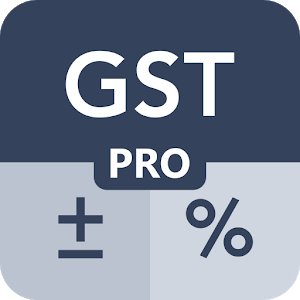Site last updated on (Today)Rating: 4.2/5

Calculate GST(Goods and Service Tax) and Percentage based calculations along with other mathematical calculations using this GST Calculator Pro Tool. Combination of Combined GST Calculator with General Calculator alongside an exclusive Simple GST Calculator is a easy to use combo a quick way to calculate Goods or Service Tax on purchase or sales of Goods or Services.

## Combined GST Calculator

Combination of GST Calculator and General Purpose Calculator, this calculator is useful to calculate GST along with general mathematical calculations like adding, subtracting, multiplying and dividing. Likewise any general calculator you can also carry custom percentage based calculations and carry out memory based calculations using M+, M-, MR and GT.

### » Features

» Embeded GST Calculator with General Purpose Calculator
» Easily and quickly Add or Remove GST from any given amount
» GST bifurcation can be seen in GST Calculation Box when clicked on GST Rates
» Addition, Subtraction, Multiplication, Division and percentage based calculations
» Upto 20 digit longer calculations
» M+, M-, MR and GT functionalities
» Customize tax rates as per requirement
» Watch what you calculate with a scrollable calculation view

## Exclusive Simple GST Calculator

Calculate GST on purchase or saled of goods or services quickly with simple user interface optimised specially for GST calculations.

To calculate GST using this calculator tool you simply need to enter the initial amount of any transaction, good or service and the rate of GST applicable to that. You get the GST amount and the amount inclusive of GST (using ‘Add GST’) or exclusive of GST (using ‘Remove GST’) as the result.

### » Features

» Add or Remove GST from given amount with touch of a button
» Set custom tax rate along with preprovided 3%, 5%, 12%, 18% and 28%
» Get results calculated as you type in
» Shows, copy, save and share IGST, CGST and SGST bifurcations
» Upto 20 digit longer calculations
https://android.giveawayoftheday.com/gst-calculator-pro-tool/